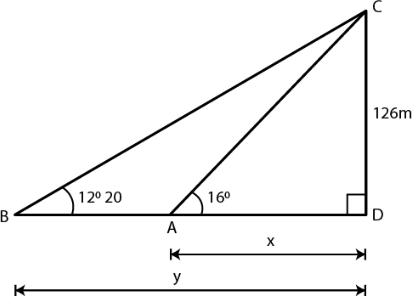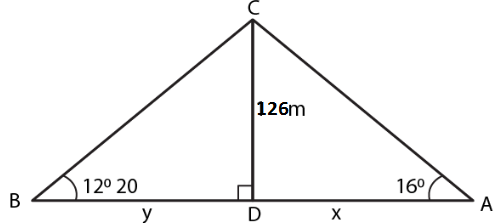Guru

# . From a tower 126 m high, the angles of depression of two rocks which are in a horizontal line through the base of the tower are 16 and 12 20’. Find the distance between the rocks if they are on (i) the same side of the tower (ii) the opposite sides of the tower.

• 0

sir this is the important  question from the book -ML aggarwal( avichal publication) class 10th , chapter20 , heights and distances

From a tower 126 m high,
the angles of depression of two rocks which are in a horizontal line through the base of the tower are 16 and 12 20’.
Find the distance between the rocks if they are on
(i) the same side of the tower
(ii) the opposite sides of the tower.

question no 30 , heights and distances , ICSE board

Share

1. Consider CD as the tower of height = 126 m

A and B are the two rocks on the same line

Angles of depression are 160 and 120 20’Substituting the values

tan 160 = 126/x

0.2867 = 126/x

So we get

x = 126/0.2867

x = 439.48

In right triangle CBD

tan 120 20’ = 126/y

So we get

0.2186 = 126/y

y = 126/0.2186 = 576.40

(i) In the first case

On the same side of the tower

AB = y – x

Substituting the values

AB = 576.40 – 439.48

AB = 136.92 m

(ii) In the second case

On the opposite side of the tower